International Fixed Income Topic IVA International Fixed Income

• Slides: 20International Fixed Income Topic IVA: International Fixed Income Pricing HedgingOutline • • Review of foreign bond investments Hedging and its consequences Empirical evidence Concluding remarksI. Review: Rates of Return on Bonds Consider a a foreign government bond. What is it’s US \$ rate of return? Taking logs of the above and rearranging gives us This is approximately equal to: [yield] - [dur x (Dr)] - %DS(Fn/\$)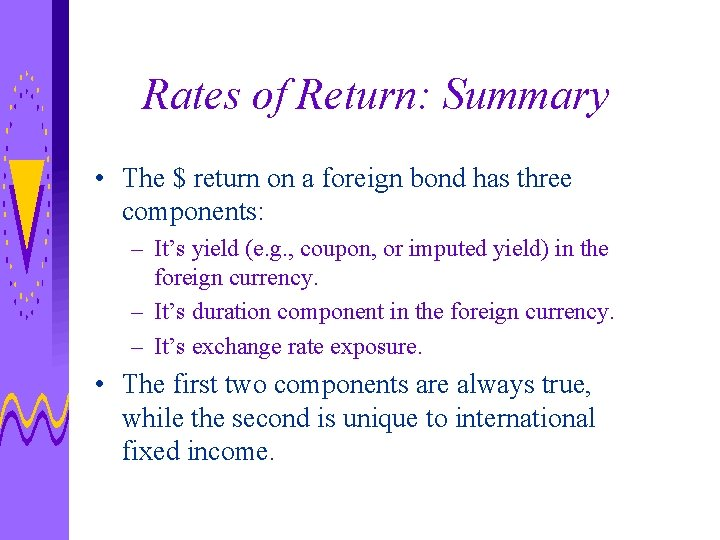Rates of Return: Summary • The \$ return on a foreign bond has three components: – It’s yield (e. g. , coupon, or imputed yield) in the foreign currency. – It’s duration component in the foreign currency. – It’s exchange rate exposure. • The first two components are always true, while the second is unique to international fixed income.Rates of Return: Summary Continued. . . • The risk associated with this return can be broken up into two pieces: – interest rate risk (i. e. , duration and maybe convexity) as the first component (i. e. , the coupon) is fixed. – exchange rate risk. Of course, if there is no exchange rate risk, we just get the usual result that the volatility of a bond is its duration times the volatility of rates.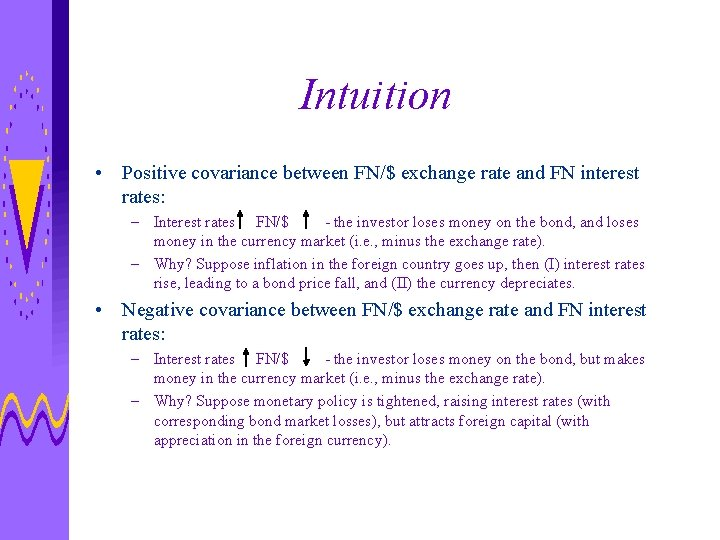Intuition • Positive covariance between FN/\$ exchange rate and FN interest rates: – Interest rates FN/\$ - the investor loses money on the bond, and loses money in the currency market (i. e. , minus the exchange rate). – Why? Suppose inflation in the foreign country goes up, then (I) interest rates rise, leading to a bond price fall, and (II) the currency depreciates. • Negative covariance between FN/\$ exchange rate and FN interest rates: – Interest rates FN/\$ - the investor loses money on the bond, but makes money in the currency market (i. e. , minus the exchange rate). – Why? Suppose monetary policy is tightened, raising interest rates (with corresponding bond market losses), but attracts foreign capital (with appreciation in the foreign currency).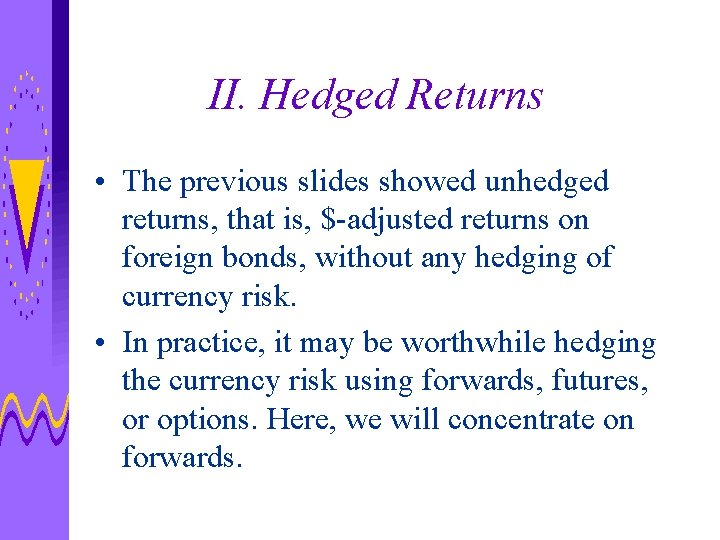II. Hedged Returns • The previous slides showed unhedged returns, that is, \$-adjusted returns on foreign bonds, without any hedging of currency risk. • In practice, it may be worthwhile hedging the currency risk using forwards, futures, or options. Here, we will concentrate on forwards.Hedging Mathematics Recall the unhedged return on \$-adjusted foreign bonds: Two strategies: (I) Buy foreign bond at price P, and sell all future coupon and principal payments in exchange for \$. (This is like a currency swap, and transforms the foreign bond into a \$ bond via interest rate parity). We will see that next class. (II) Alternatively, sell a one-period forward currency contract equal to next period’s estimated value of the bond (plus interest if any).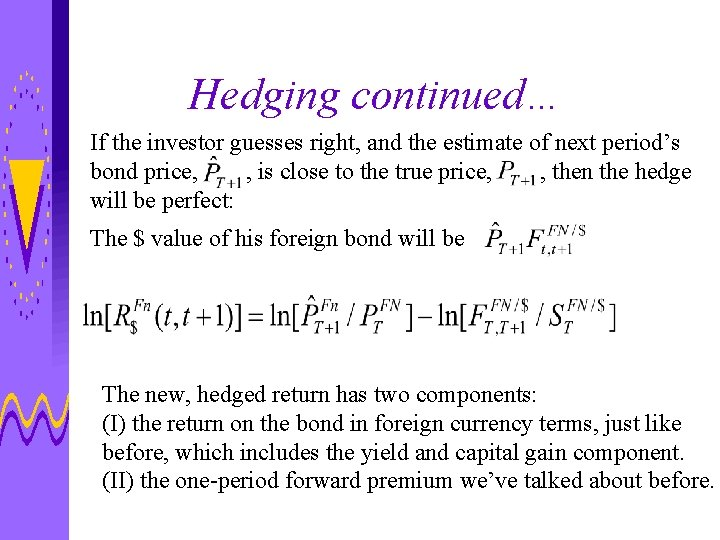Hedging continued… If the investor guesses right, and the estimate of next period’s bond price, , is close to the true price, , then the hedge will be perfect: The \$ value of his foreign bond will be The new, hedged return has two components: (I) the return on the bond in foreign currency terms, just like before, which includes the yield and capital gain component. (II) the one-period forward premium we’ve talked about before.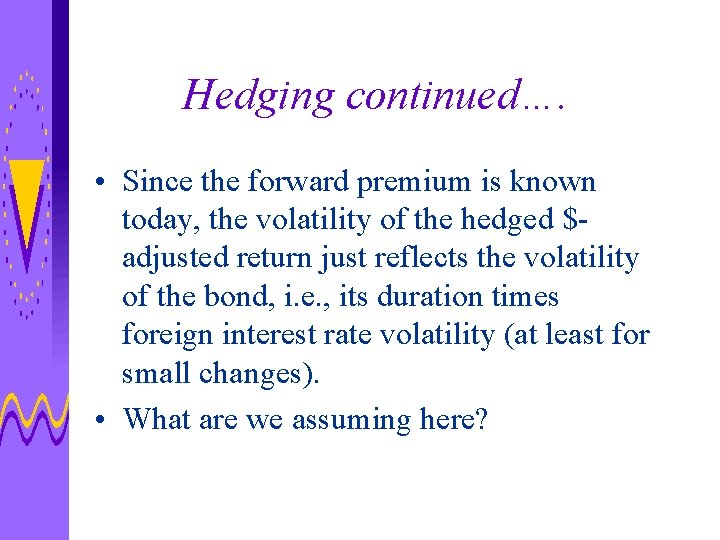Hedging continued…. • Since the forward premium is known today, the volatility of the hedged \$adjusted return just reflects the volatility of the bond, i. e. , its duration times foreign interest rate volatility (at least for small changes). • What are we assuming here?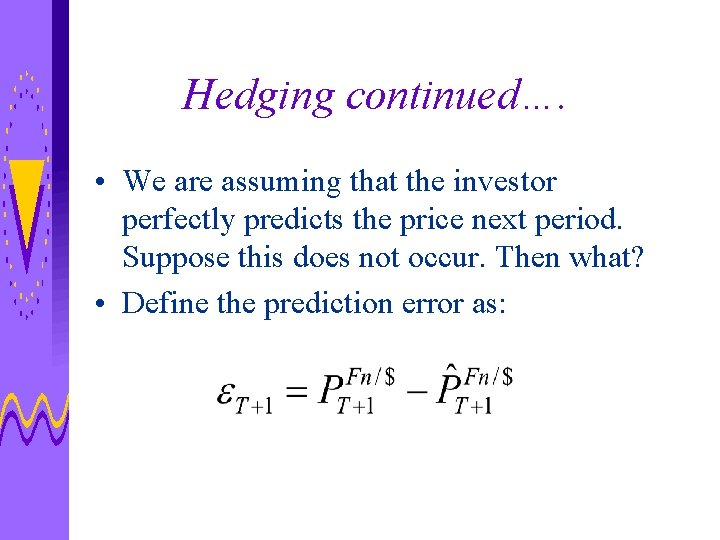Hedging continued…. • We are assuming that the investor perfectly predicts the price next period. Suppose this does not occur. Then what? • Define the prediction error as:Hedging continued…. • If e was too large, then our hedge amount was too small and the unexpected excess value of the bond is valued at St+1. If e was too small, then our hedge was too large and we need to buy additional funds at at St+1. • We can therefore measure the return on the foreign bond in \$, hedged but not perfectly….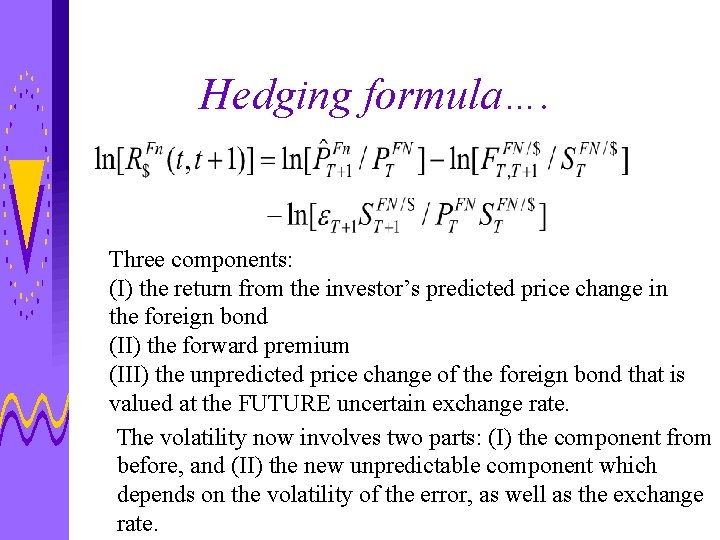Hedging formula…. Three components: (I) the return from the investor’s predicted price change in the foreign bond (II) the forward premium (III) the unpredicted price change of the foreign bond that is valued at the FUTURE uncertain exchange rate. The volatility now involves two parts: (I) the component from before, and (II) the new unpredictable component which depends on the volatility of the error, as well as the exchange rate.III. Empirical Evidence • Two cases: – Monthly data; 10 year-period during 1980’s; G 7 countries, plus global portfolio. – Weekly data; 1996 -1999; G 7 countries, as previous lectures. • Comparison of unhedged and hedged returns.Mean \$-adjusted Annualized Return on Foreign Bond Portfolios (1980’s)Volatility of \$-adjusted Annualized Return on Foreign Bond Portfolios (1980’s)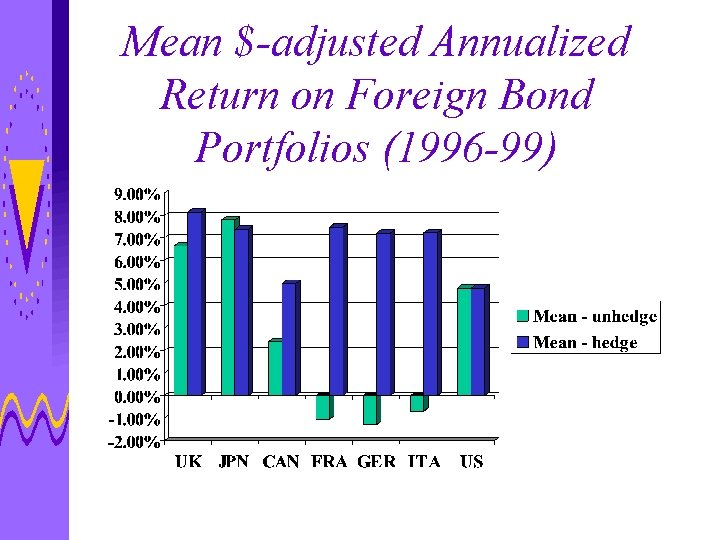Mean \$-adjusted Annualized Return on Foreign Bond Portfolios (1996 -99)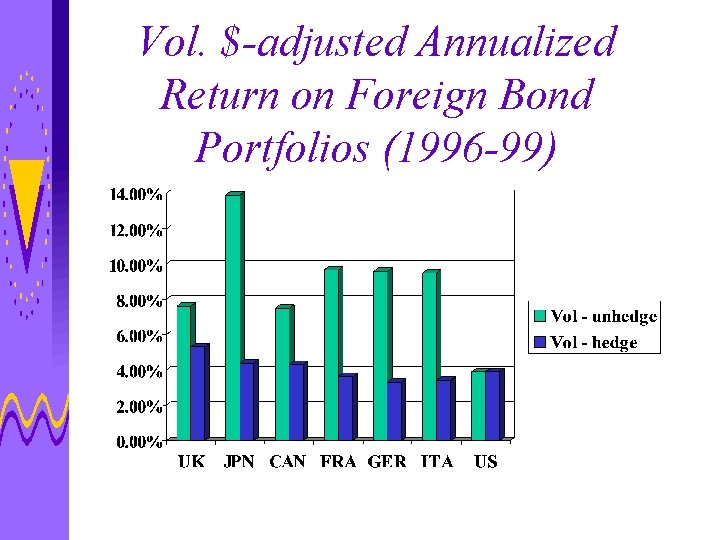Vol. \$-adjusted Annualized Return on Foreign Bond Portfolios (1996 -99)Recall: % of Volatility of \$-adjusted Foreign Bond Due to Currency Risk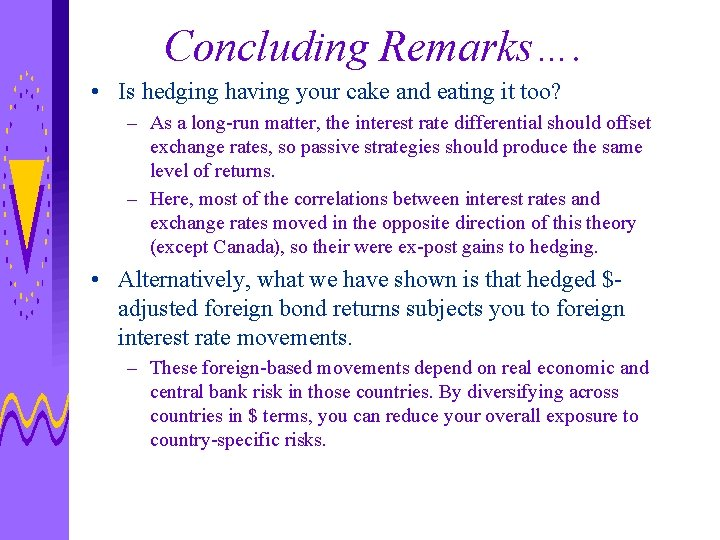Concluding Remarks…. • Is hedging having your cake and eating it too? – As a long-run matter, the interest rate differential should offset exchange rates, so passive strategies should produce the same level of returns. – Here, most of the correlations between interest rates and exchange rates moved in the opposite direction of this theory (except Canada), so their were ex-post gains to hedging. • Alternatively, what we have shown is that hedged \$adjusted foreign bond returns subjects you to foreign interest rate movements. – These foreign-based movements depend on real economic and central bank risk in those countries. By diversifying across countries in \$ terms, you can reduce your overall exposure to country-specific risks.